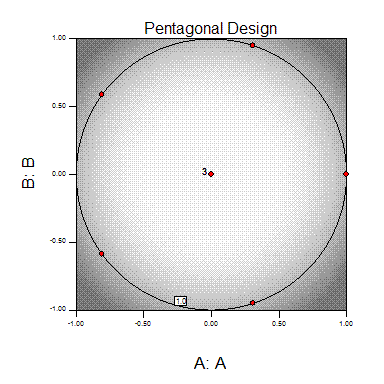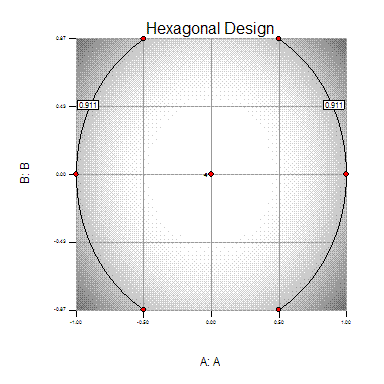# Miscellaneous¶

A set of catalog designs with preselected points to estimate quadratic models.

We recommend using more standard designs such as the central composite or Box-Behnken.

## 3-Level Factorial Design¶

In Design-Expert, these designs are located under the Response Surface, Miscellaneous design node. Full factorial 3-level designs are available for up to 4 factors. The number of experiments will be 3^k plus some replicates of the center point. Because there are only 3 levels for each factor, the appropriate model is the quadratic model. For more than 2 factors, these designs force you to run many more experiments than are needed to estimate the coefficients in a quadratic model. A Box-Behnken design also requires only three-levels, and is a more efficient alternative to the full three-level factorial.

Factors Model Terms Number of Runs
2 6 13
3 10 32
4 15 87

You may add up to ten categoric factors to this design. This will cause the number of runs generated to be multiplied by the number of combinations of the categorical factor levels. In this case, you may prefer an optimal design.

Three-level factorial designs may be carried out in blocks. These designs can be run in one block or split into three blocks of equal size.

## Hybrid Design¶

The hybrid designs in Design-Expert software are from Roquemore’s paper [Roq76].

They are hard coded in our design catalog; i.e. the design points are read in, not generated. The particular 3, 4 and 6 factor designs are D311A, D416B and D628A. If you sort the design by standard order you get the designs layout in Roquemore’s paper. The 7 factor design was not named but its method of construction is also in the paper.

In each case the first K-1 columns are from a CCD and their factorial points are at +/- 1 and the axial values are those with values greater than 1. The last column does not have the form of a column for a CCD; i.e. having factorial and axial values.

However, the hybrid design will be sensitive to outliers or missing data. It should be used ONLY when budget considerations prohibit use of a regular CCD, Box-Behnken or full three-level factorial design AND you are certain that all the runs can be performed. For more details on the construction of a hybrid design see Myers and Montgomery, 1995 [MM95].

## Pentagonal Design¶

For two factors the pentagonal design is an interesting geometrically spaced design that may be useful in particular situations. The pentagon has one apex at (1, 0). There are 4 levels of factor A and 5 levels of factor B.

There are five model terms in the quadratic model for two factors - A, B, AB, A2 , B2 - and only five points plus a replicated center point in this design, so this is a minimal point design. The five pentagonal points have a leverage of 1.0, this design is extremely sensitive to outliers. More points should be added to assess lack of fit. You may also add categoric factors to this design. This will cause the number of runs generated to be multiplied by the number of combinations of the categoric factor levels. Basically, you will run the pentagonal design at every combination of categoric factor levels.## Hexagonal Design¶

For two factor designs the hexagonal design is an interesting geometrically spaced design that may be useful in particular situations. There are 5 levels of factor A and 3 levels of factor B. This design is slightly better than the pentagonal design, in that there are no points with leverage 1.0. You may also add categoric factors to this design. This will cause the number of runs generated to be multiplied by the number of combinations of the categoric factor levels. Basically, you will run the hexagonal design at every combination of categoric factor levels.References

 [MM95] Raymond H. Meyers and Douglas C. Montgomery. Response Surface Methodology: Process and Product in Optimization Using Designed Experiments. Wiley, 1995.
 [Roq76] K. G. Roquemore. Hybrid designs for quadratic response surfaces. Technometrics, 18(4):419–423, 1976.